# Determination of cross section size of each component of casting parts of support platform

The section size of each component can be obtained by simple empirical formula according to the type, quality, size, wall thickness and casting time of casting alloy.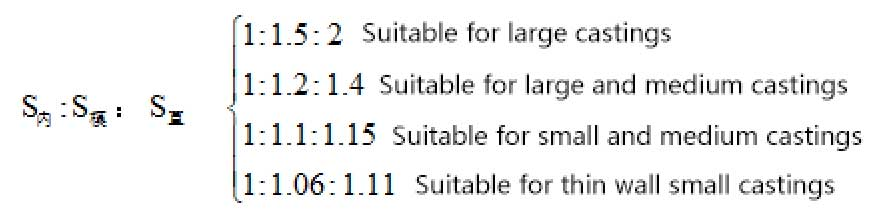In production, the minimum cross-sectional area of the internal sprue is 0.04cm2, and the minimum diameter of the direct sprue is generally not less than 15-18mm.

Calculation formula of flow resistance section of gray cast iron: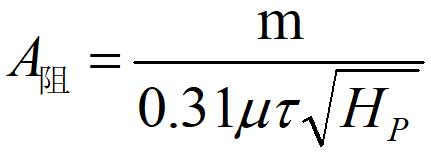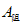——Total area of the minimum section in the gating system (cm2);

M — total weight of liquid metal flowing through f resistance section (kg);

μ – total flow loss coefficient;

In the formula, M is 8.6kg; μ = 0.42 ;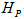= 110cm;

The pouring time is calculated as follows: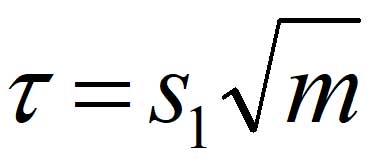M – total mass (weight) of metal liquid in the mold (kg)—Coefficient, depending on the wall thickness of the casting, it is found from the table that = 2.2 .

The pouring time is 6.5s.

As the parts of the support table are small and medium-sized castings, the section ratio is determined to be 1:1.1:1.15.

According to the calculation formula of the flow resistance section, the cross-sectional area of the internal sprue is 8.90, and it is found from the table that a = 56mm, B = 52mm

, C = 17mm; section area of sprue = 10.24, d = 18mm according to the table; section area of transverse sprue = 9.97, a = 32mm, B = 22mm, C = 35mm. Therefore, the schematic diagram of each sprue of the supporting platform parts is as follows: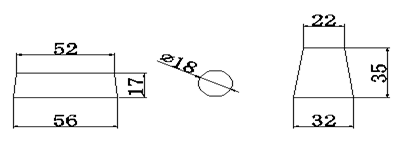Sectional area of internal sprue, direct sprue and cross sprue

Table 1 standard values of gating system for gray iron castings

 Internal sprue size/mm （S(in)/m㎡ ) Runner size/mm （S(tr)/m㎡ Sprue size/mm （S(str)/ m㎡)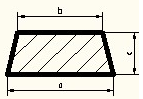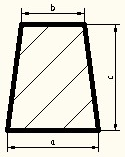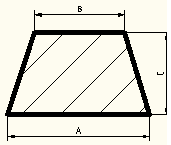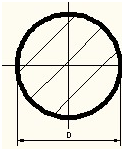a b c S(in) a b c S(in) A B C S(tr) D S(str) 11 9 5 50 6 4 10 50 16 11 18 240 17 230 14 12 6 80 8 5 12 80 19 14 22 360 20 310 18 15 7 115 10 6 15 120 23 15 25 480 23 420 20 18 8 150 11 7 17 150 28 18 31 720 27 570 24 21 10 225 13 9 21 225 32 22 35 950 32 800 30 26 11 310 14 10 26 310 38 28 42 1380 38 1130 40 30 12 455 17 11 33 450 46 32 50 1950 45 1590 45 41 14 600 20 12 37 600 56 40 58 2800 53 2200 56 52 17 920 24 16 46 920 65 45 70 3850 65 3320 58 53 22 1200 28 20 50 1200 80 60 80 5600 80 5030

Riser and size determination

Generally small castings with uniform wall thickness may not have riser, so it is omitted here.